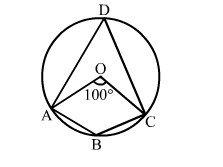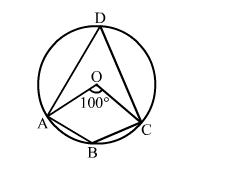# In the given figure, O is the centre of the given circle and measure of arc ABC is 100°.

Question:

In the given figure, O is the centre of the given circle and measure of arc ABC is 100°. Determine ∠ADC and ∠ABC.Solution:We know that the angle subtended by an arc is twice the angle subtended by it on the circumference in the alternate segment.